# Edge detection using Prewitt, Scharr and Sobel Operator

• Last Updated : 16 Oct, 2021

The discontinuity in the image brightness is called an edge. Edge detection is the technique used to identify the regions in the image where the brightness of the image changes sharply. This sharp change in the intensity value is observed at the local minima or local maxima in the image histogram, using the first-order derivative.

The techniques used here in this article are first-order derivative techniques namely:

## Prewitt Operator

The Prewitt operator was developed by Judith M. S. Prewitt. Prewitt operator is used for edge detection in an image. Prewitt operator detects both types of edges, these are:

• Horizontal edges or along the x-axis,
• Vertical Edges or along the y-axis.

Wherever there is a sudden change in pixel intensities, an edge is detected by the mask. Since the edge is defined as the change in pixel intensities, it can be calculated by using differentiation. Prewitt mask is a first-order derivate mask. In the graph representation of Prewitt-mask’s result, the edge is represented by the local maxima or local minima.

•  More weight means more edge detection.
•  The opposite sign should be present in the mask. (+ and -)
•  The Sum of the mask values must be equal to zero.

Prewitt operator provides us two masks one for detecting edges in the horizontal direction and another for detecting edges in a vertical direction.

Prewitt Operator [X-axis] = [ -1 0 1; -1 0 1; -1 0 1]

Prewitt Operator [Y-axis] = [1 1 1; 1 1 1; 1 1 1]

Steps:

• Convert into grayscale if it is colored.
• Convert into the double format.
• Define the mask or filter.
• Detect the edges along X-axis.
• Detect the edges along Y-axis.
• Combine the edges detected along the X and Y axes.
• Display all the images.

Imtool() is the inbuilt function in Matlab. It is used to display the image. It takes 2 parameters; the first is the image variable and the second is the range of intensity values. We provide an empty list as the second argument which means the complete range of intensity has to be used while displaying the image.

Example:

## Matlab

 `% MATLAB code for prewitt``% operator edge detection``k=imread(``"logo.png"``);``k=rgb2gray(k);``k1=double(k);``p_msk=[-1 0 1; -1 0 1; -1 0 1];``kx=conv2(k1, p_msk, ``'same'``);``ky=conv2(k1, p_msk``', '``same');``ked=sqrt(kx.^2 + ky.^2);` `% display the images.``imtool(k,[]);`` ` `% display the edge detection along x-axis.``imtool(abs(kx), []);` `% display the edge detection along y-axis.``imtool(abs(ky),[]);`` ` `% display the full edge detection.``imtool(abs(ked),[]);`

Output: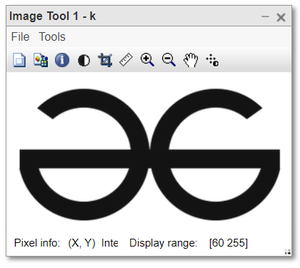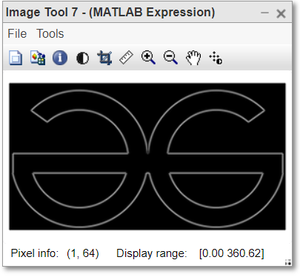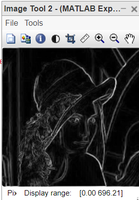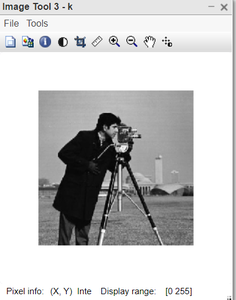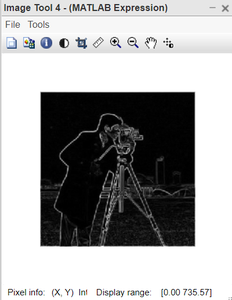## Scharr Operator

This is a filtering method used to identify and highlight gradient edges/features using the first derivative. Performance is quite similar to the Sobel filter.

Scharr Operator [X-axis] = [-3 0 3; -10 0 10; -3 0 3];

Scharr Operator [Y-axis] = [ 3 10 3; 0 0 0; -3 -10 -3];

Example:

## Matlab

 `%Scharr operator -> edge detection`` ``k=imread(``"logo.png"``);`` ``k=rgb2gray(k);`` ``k1=double(k);`` ``s_msk=[-3 0 3; -10 0 10; -3 0 3];`` ``kx=conv2(k1, s_msk, ``'same'``);`` ``ky=conv2(k1, s_msk``', '``same');`` ``ked=sqrt(kx.^2 + ky.^2);`` ``%display the images.`` ``imtool(k,[]);`` ``%display the edge detection along x-axis.`` ``imtool(abs(kx), []);`` ``%display the edge detection along y-axis.`` ``imtool(abs(ky),[]);`` ``%display the full edge detection.`` ``imtool(abs(ked),[]);`

Output: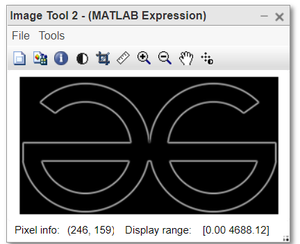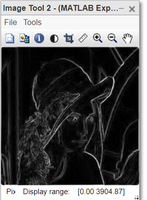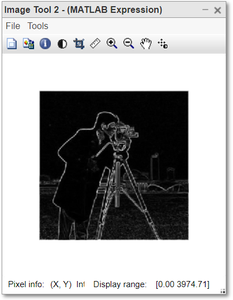## Sobel Operator

It is named after Irwin Sobel and Gary Feldman. Like the Prewitt operator Sobel operator is also used to detect two kinds of edges in an image:

• Vertical direction
• Horizontal direction

The difference between Sobel and Prewitt Operator is that in Sobel operator the coefficients of masks are adjustable according to our requirement provided they follow all properties of derivative masks.

Sobel-X Operator = [-1 0 1; -2 0 2; -1 0 1]

Sobel-Y Operator = [-1 -2 -1; 0 0 0; 1 2 1]

Example:

## Matlab

 `% MATLAB code for Sobel operator``% edge detection`` ``k=imread(``"logo.png"``);`` ``k=rgb2gray(k);`` ``k1=double(k);`` ``s_msk=[-1 0 1; -2 0 2; -1 0 1];`` ``kx=conv2(k1, s_msk, ``'same'``);`` ``ky=conv2(k1, s_msk``', '``same');`` ``ked=sqrt(kx.^2 + ky.^2);``  ` ` ``%display the images.`` ``imtool(k,[]);``  ` ` ``%display the edge detection along x-axis.`` ``imtool(abs(kx), []);``  ` ` ``%display the edge detection along y-axis.`` ``imtool(abs(ky),[]);``  ` ` ``%display the full edge detection.`` ``imtool(abs(ked),[]);`` `

Output: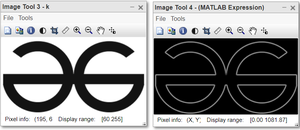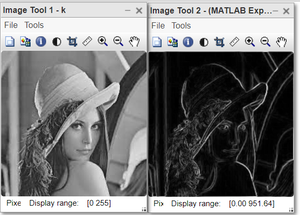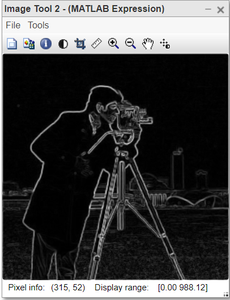My Personal Notes arrow_drop_up